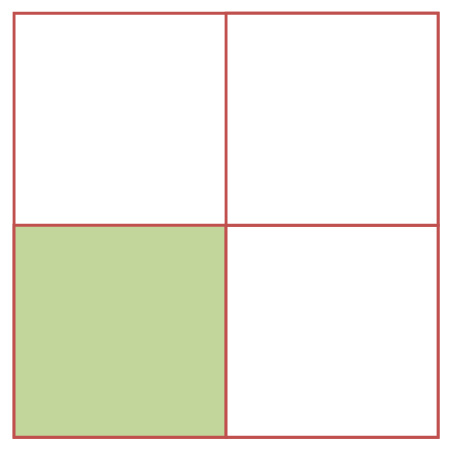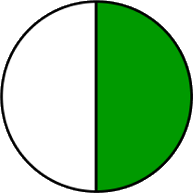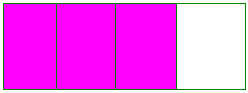3rd grade Division
3rd grade Mental Math
3rd grade Problem Solving
3rd grade Fractions
3rd grade multiplication
100

40 divided by 5 =

8

100

20+50

70

100

Using coins show 2 different ways to make 25 cents.

Either:

* 25 pennies

* 5 nickels

* 2 dimes & 5 pennies

* 2 dimes and 1 nickel

* 1 quarter

100

Draw a square and shade in one-forth.100
9x9

81

200

60 divided by ______ = 10

6

200

55+55

110

200

Sue swims in the pool from 1:10pm to 1:35pm. For how many minutes did she swim?

25 minutes

200

Draw a circle and shade in one-half.200

15x21

315

300

54 divided by 9 =_______

6

300

120+120

240

300

Mark is 17, Jill is 13.  What's the DIFFERENCE in their ages?

17-13=4

300

Draw a rectangle and shade in three fourths.300

8x7

56

400

________ divided by 4 = 6

24

400

400+600

1000

400

If James has 40 books and 10 empty book shelves. He wants to put an even number of books on each shelf and wants to use all 40 books. How many books will go on each shelf?

4

400

Which has a larger value 1/2 or 1/4?

1/2

400

12x5

60

500

32 divided by ______ = 8

4

500

1100+1100

2200

500

There are 3 pigs and 2 chickens. How many legs are there all together?

3*4= 12

2*2= 4

12+4= 16

16 legs all together

500

What is the total value of 1/2 plus 1/2?

1

500

12x12

144

Click to zoom